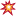Pour poster sur ce forum, tu dois t'identifierpilato  (19.05.2022 à 21:10)

Photo 1 = 4
Photo 2 = 7
Photo 3 = 199
Photo 4 = 27
Photo 5 = 401
Photo 6 = 402+1=403

Total = 1041

Page  53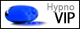Supersympa  (20.05.2022 à 18:16)

Photo 1 = 4
Photo 2 = 7
Photo 3 = 199
Photo 4 = 27
Photo 5 = 401
Photo 6 = 403 + 1 = 404

Total = 1042

Page  53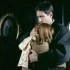Photo du mois 2sanct08  (20.05.2022 à 21:10)

Photo 1 = 4
Photo 2 = 7
Photo 3 = 199+1=200
Photo 4 = 27
Photo 5 = 401
Photo 6 = 404

Total = 1043

Page  53pilato  (20.05.2022 à 23:43)

Photo 1 = 4
Photo 2 = 7
Photo 3 = 200+1=2001
Photo 4 = 27
Photo 5 = 401
Photo 6 = 404

Total = 1044

Page  53Rebekkah12  (21.05.2022 à 00:30)

Photo 1 = 4
Photo 2 = 7
Photo 3 = 201
Photo 4 = 27
Photo 5 = 401+1=402
Photo 6 = 404

Total = 1045

Page  53Photo du mois 2sanct08  (21.05.2022 à 11:54)

Photo

1 = 4
Photo 2 = 7
Photo 3 = 201
Photo 4 = 27
Photo 5 = 402+1=403
Photo 6 = 404

Total = 1046

Page  53Supersympa  (21.05.2022 à 14:53)

Photo

1 = 4
Photo 2 = 7
Photo 3 = 201
Photo 4 = 27
Photo 5 = 403
Photo 6 = 404 + 1 = 405

Total = 1047

Page  53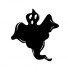Luna25  (21.05.2022 à 22:16)

Photo 1 = 4
Photo 2 = 7
Photo 3 = 201+1=202
Photo 4 = 27
Photo 5 = 403
Photo 6 = 405

Total = 1048

Page  53pilato  (21.05.2022 à 22:49)

Photo 1 = 4
Photo 2 = 7
Photo 3 = 202+1=203
Photo 4 = 27
Photo 5 = 403
Photo 6 = 405

Total = 1049

Page  53Supersympa  (22.05.2022 à 10:10)

Photo 1 = 4
Photo 2 = 7
Photo 3 = 203
Photo 4 = 27
Photo 5 = 403
Photo 6 = 405 + 1 = 406

Total = 1050

Page  53pilato  (22.05.2022 à 20:26)

Photo 1 = 4
Photo 2 = 7
Photo 3 = 203
Photo 4 = 27
Photo 5 = 403
Photo 6 = 406+1=407

Total = 1051

Page  53Rebekkah12  (22.05.2022 à 23:01)

Photo 1 = 4
Photo 2 = 7
Photo 3 = 203
Photo 4 = 27
Photo 5 = 403+1=404
Photo 6 = 407

Total = 1052

Page  53Photo du mois 2sanct08  (23.05.2022 à 13:16)

Photo 1 = 4
Photo 2 = 7
Photo 3 = 203
Photo 4 = 27
Photo 5 = 404+1=405
Photo 6 = 407

Total = 1053

Page  53mnoandco  (23.05.2022 à 13:19)

Photo 1 = 4
Photo 2 = 7
Photo 3 = 203+1=204
Photo 4 = 27
Photo 5 = 405
Photo 6 = 407

Total = 1054

Page  53Supersympa  (23.05.2022 à 16:36)

Photo 1 = 4
Photo 2 = 7
Photo 3 = 204
Photo 4 = 27
Photo 5 = 405
Photo 6 = 407 + 1 = 408

Total = 1055

Page  53pilato  (23.05.2022 à 22:08)

Photo 1 = 4
Photo 2 = 7
Photo 3 = 204
Photo 4 = 27
Photo 5 = 405
Photo 6 = 408+1=409

Total = 1056

Page  53Rebekkah12  (23.05.2022 à 22:45)

Photo 1 = 4
Photo 2 = 7
Photo 3 = 204
Photo 4 = 27
Photo 5 = 405+1=406
Photo 6 = 409

Total = 1057

Page  53Photo du mois 2sanct08  (24.05.2022 à 12:10)

Photo 1 = 4
Photo 2 = 7
Photo 3 = 204
Photo 4 = 27
Photo 5 = 406+1=407
Photo 6 = 409

Total = 1058

Page  53pilato  (24.05.2022 à 16:35)

Photo 1 = 4
Photo 2 = 7
Photo 3 = 204
Photo 4 = 27
Photo 5 = 407+1=408
Photo 6 = 409

Total = 1059

Page  53Rebekkah12  (24.05.2022 à 16:53)

Photo 1 = 4
Photo 2 = 7
Photo 3 = 204
Photo 4 = 27
Photo 5 = 408+1=409
Photo 6 = 409

Total = 1060

Page  53Supersympa  (24.05.2022 à 19:12)

Photo 1 = 4
Photo 2 = 7
Photo 3 = 204
Photo 4 = 27
Photo 5 = 409
Photo 6 = 409 + 1 = 410

Total = 1061

Page  53Luna25  (24.05.2022 à 21:51)

Photo 1 = 4
Photo 2 = 7
Photo 3 = 204+1=205
Photo 4 = 27
Photo 5 = 409
Photo 6 = 410

Total = 1062

Page  53mnoandco  (25.05.2022 à 14:16)

Photo 1 = 4
Photo 2 = 7
Photo 3 = 205
Photo 4 = 27
Photo 5 = 409+1=410
Photo 6 = 410

Total = 1063

Page  53pilato  (25.05.2022 à 16:05)

Photo 1 = 4
Photo 2 = 7
Photo 3 = 205
Photo 4 = 27
Photo 5 = 410+1=411
Photo 6 = 410

Total = 1064

Page  53Supersympa  (25.05.2022 à 18:01)

Photo 1 = 4
Photo 2 = 7
Photo 3 = 205
Photo 4 = 27
Photo 5 = 411
Photo 6 = 410 + 1 = 411

Total = 1065

Page  53Rebekkah12  (25.05.2022 à 22:51)

Photo 1 = 4
Photo 2 = 7
Photo 3 = 205
Photo 4 = 27
Photo 5 = 411+1=412
Photo 6 = 411

Total = 1066

Page  53mnoandco  (26.05.2022 à 12:41)

Photo 1 = 4
Photo 2 = 7
Photo 3 = 205
Photo 4 = 27
Photo 5 = 412
Photo 6 = 411+1=412

Total = 1067

Page  53Supersympa  (26.05.2022 à 14:08)

Photo 1 = 4
Photo 2 = 7
Photo 3 = 205
Photo 4 = 27
Photo 5 = 412
Photo 6 = 412 + 1 = 413

Total = 1068

Page  53StoneHeart  (26.05.2022 à 15:58)

Photo 1 = 4
Photo 2 = 7
Photo 3 = 205
Photo 4 = 27
Photo 5 = 412+1=413
Photo 6 = 413

Total = 1069

Page  53Rebekkah12  (26.05.2022 à 22:41)

Photo 1 = 4
Photo 2 = 7
Photo 3 = 205
Photo 4 = 27
Photo 5 = 413+1=414
Photo 6 = 413

Total = 1070

Page  53

Ne manque pas...
Activité récente
Actualités

Une deuxième fille pour Stephen Amell !
A la surprise générale, Stephen Amell et Cassandra Jean ont agrandi leur famille ! Déjà parents de...

La franchise "Arrowverse" en lice sur Le Caméléon
Le quartier Le Caméléon  vient de lancer son nouveau sondage qui vous propose de départager des...

Calendrier du mois !
Le calendrier du mois de mars 2022 est arrivé sur le quartier et nous est proposé par serieserie !...

Calendrier du mois !
Le calendrier du mois de février 2022 est arrivé sur le quartier et nous est proposé par serieserie...

Les nouveautés des séries et de notre site une fois par mois dans ta boîte mail ?

HypnoRooms

bloom74, 21.06.2022 à 07:34

Bonjour, dernier jour pour voter pour la manche2 de la SuperBattle du quartier The Boys. A vous de jouer.

KylianM, 21.06.2022 à 17:12

Venez récompenser les séries quotidiennes françaises avec Les Quotidiennes Awards sur le quartier de Plus belle la vie !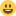CastleBeck, 22.06.2022 à 11:27

Le survivor du quartier This Is Us compte sur vos votes! MerciShanInXYZ, 22.06.2022 à 17:10

Nouveau thème dans Voyage au centre du Tardis, quelle photo de Dan Lewis allez vous nous dénicher ? Passez voir le Docteur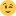bloom74, 22.06.2022 à 17:34

Et voilà la 3e Manche de la SuperBattle est en cours, les combats de titans ont commencé. Retrouvez les sur le quartier The Boys !